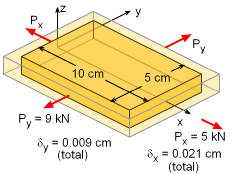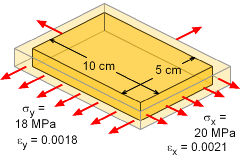Ch 1. Stress and Strain Multimedia Engineering Mechanics NormalStress Shear andBearing Stress NormalStrain Hooke'sLaw ThermalEffects IndeterminateStructures
 Chapter 1. Stress/Strain 2. Torsion 3. Beam Shr/Moment 4. Beam Stresses 5. Beam Deflections 6. Beam-Advanced 7. Stress Analysis 8. Strain Analysis 9. Columns Appendix Basic Math Units Basic Equations Sections Material Properties Structural Shapes Beam Equations Search eBooks Dynamics Fluids Math Mechanics Statics Thermodynamics Author(s): Kurt Gramoll ©Kurt GramollMECHANICS - EXAMPLE ExamplePlate with Applied Loads and Displacement A 0.5 cm thick rectangular plate is pulled in tension by two loads in the x and y directions as shown. The total deflection in the x and y direction is 0.021 cm and 0.009 cm, respectively. What is the Poisson's ratio of the material? The z direction has no load and the deflection is not known. SolutionStresses and Strains on Plate This problem involves loading from two directions, and thus requires at least the 2-D Hooke's Law. The 3-D Hooke's Law could be used, but since σz is zero, those equations will reduce to the 2-D equations. The equations are,      εx = (σx - ν σy)/E      εy = (σy - ν σx)/E The strains and stresses in the x and y direction need to be calculated.      σx = Px/Ax = 5/[(0.05)(0.005)] = 20 MPa      σy = Py/Ay = 9/[(0.10)(0.005)] = 18 MPa      εx = 0.021/10 = 0.0021 cm/cm      εy = 0.009/5 = 0.0018 cm/cm Substituting the stresses and strains into the 2-D Hooke's Law equations, gives      0.0021 E = 20 - ν 18      0.0018 E = 18 - ν 20 Solving for ν gives,      ν = 0.1876

Practice Homework and Test problems now available in the 'Eng Mechanics' mobile app
Includes over 400 problems with complete detailed solutions.
Available now at the Google Play Store and Apple App Store.